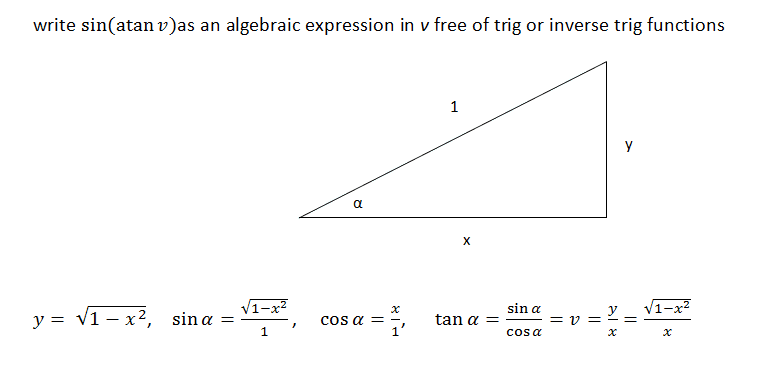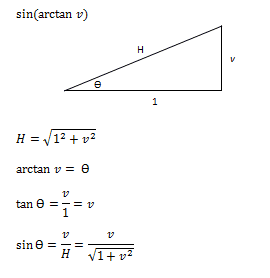# Write the trigonometric expression as an algebraic expression

jkristia

## Homework Statement

Not long ago I had a similar problem, which I was able to solve after reading this thread, but for this question I'm stuck and I could use a small hint.Thanks

## Answers and Replies

scurty
I would first solve for $arctan(v)$ in terms of $\alpha$. See if you can do the rest!

Staff Emeritus
Homework Helper
Gold Member

## Homework Statement

Not long ago I had a similar problem, which I was able to solve after reading this thread, but for this question I'm stuck and I could use a small hint.## The Attempt at a Solution

Thanks
Label the triangle differently. Label it so that the tangent of α is v .

$\displaystyle \tan(\alpha)=\frac{v}{1}\,.$

jkristia
>>I would first solve for arctan(v) in terms of α

>>Label the triangle differently. Label it so that the tangent of α is v .

hmm, I think that is what I have, tan (α) = v = sqrt..., I'm still missing something.

scurty
>>I would first solve for arctan(v) in terms of α

>>Label the triangle differently. Label it so that the tangent of α is v .

hmm, I think that is what I have, tan (α) = v = sqrt..., I'm still missing something.

Okay, so you have $tan(\alpha) = v$. What is $arctan(v)$? Then, working from the inside out, what is $sin(arctan(v))$?

jkristia
That is the part I'm missing, how do I find the exact value of atan(v). I know what the result is, but I dont know how to get to it.

scurty
That is the part I'm missing, how do I find the exact value of atan(v). I know what the result is, but I dont know how to get to it.

Look in your original picture. v is equal to four quantities, which one would give you a nice value for $arctan(v)$?

jkristia
I appreciate the help and the hints, but at the moment I'm blank. I'm sure the answer is staring right at me, and it is pretty straight forward - but I just can't see it. I will think more about this during the day and hopefully tonight when I get back to it, the answer jumps right out of the picture.

jkristia
ah - I think I got it, will try later. I need to find the inverse of v = sqrt()/x.

scurty
Sorry for the late reply, I took a nap!

What is $arctan(tan(x))$ equal to?

Can you see which value to plug in now?

Then you need to calculate sine of that number you get, which is also listed in the picture as a number not involving trig functions or trig inverses.

jkristia
I have not been able to figure this out by myself, I kept going in circles. Finally when I was about to give up I Bing'ed it and found this explanation http://mathforum.org/library/drmath/view/53946.html - and now I can see how simple it is, and I can see my main problem was the labeling as was pointed out in one of the answers. Had I labeled the figure correct, then I might have been able to figure it out on my own.

scurty
Yeah, I've had plenty of those moments, and then it just clicks. Just so we all know you understand it correctly, what answer did you get?

jkristia
This is how I solved it. It is exactly the same steps as on the link. I read the explanation, closed the browser and solved it myself by first drawing a new trigangle with the 'correct' labels, and then it pretty much fell in place.

I think the trick is to label the triangle with the sides as v/1 = v for the inner trig function, and that is where I made the mistake.... I thinkAgain, thank you for your help.

Edit: I just noticed that the tanθ does not provide any additional information.

#### Attachments

Last edited:
scurty
That looks good to me based off of the labels of the diagram! Just wanted to make sure you figured out the problem correctly!